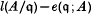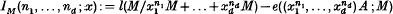Hostname: page-component-7d684dbfc8-26pbs Total loading time: 0 Render date: 2023-09-26T12:32:16.769Z Has data issue: false Feature Flags: { "corePageComponentGetUserInfoFromSharedSession": true, "coreDisableEcommerce": false, "coreDisableSocialShare": false, "coreDisableEcommerceForArticlePurchase": false, "coreDisableEcommerceForBookPurchase": false, "coreDisableEcommerceForElementPurchase": false, "coreUseNewShare": true, "useRatesEcommerce": true } hasContentIssue false

# On the dimension of the non-Cohen–Macaulay locus of local rings admitting dualizing complexes

Published online by Cambridge University Press:  24 October 2008

## Extract

In this paper we mainly consider local rings admitting dualizing complexes. It is well-known that if a Noetherian local ring A admits a dualizing complex, then the non-Cohen–Macaulay (abbreviated CM) locus of A is closed in the Zariski topology (cf. [8, 10]). If the dimension of this locus is zero and A is equidimensional, i.e. the punctured spectrum of A is locally CM and dim(A/P) = dim (A) for all minimal prime ideals P ∈ Ass (A), then A is a generalized CM ring and its structure is well-understood (see [2, 12]). For instance, one of the characterizations of generalized CM rings is the conditions that for any parameter ideal q contained in a large power of the maximal ideal m of A, the difference between length and multiplicityis independent of the choice of q. However, if the dimension of the non-CM locus is larger than zero, little is known about how this dimension is related to the structure of the local ring A. The purpose of this paper is to show that if M is a finitely generated A-module, then there exist systems of parameters x = (x1, …, xd) (where d = dim M) such that the differenceis a polynomial in n1, …, nd for all positive integers n1, …, nd and the degree of IM(n1, …, nd;x) is independent of the choice of x. We shall also give various characterizations of this degree by using the notion of reducing systems of parameters of Auslander and Buchsbaum[l]. In particular, if the module M is equidimensional we shall show that the degree of IM(n1, …, nd;x) is equal to the dimension of the non-CM locus of M.

Type
Research Article

## Access options

Get access to the full version of this content by using one of the access options below. (Log in options will check for institutional or personal access. Content may require purchase if you do not have access.)

## References

#### REFERENCES

Auslander, M. and Buchsbaum, D. A.. Codimension and multiplicity. Ann. of Math. 68 (1958), 625657.CrossRefGoogle Scholar
Cuong, N. T., Schenzel, P. and Trung, N. V.. Verallgemeinerte Cohen–Macaulay Moduln. Math. Nachr. 85 (1978), 5773.Google Scholar
Cuong, N. T.. On the length of the powers of systems of parameters in local rings. Nagoya Math. J. 120 (1990).CrossRefGoogle Scholar
Garcia Roig, J.-L.. On polynomial bounds for the Koszul homology of certain multiplicity systems. J. London Math. Soc. (2) 34 (1986), 411416.CrossRefGoogle Scholar
Grothendieck, A.. SGA II (North-Holland, 1962).Google Scholar
Hartshorne, R.. Algebraic Geometry (Springer-Verlag, 1977).CrossRefGoogle Scholar
Matsumura, H.. Commtrtatrve Algebra (Benjamin, New York, 1980).Google Scholar
Schenzel, P.. Einige Anwendungen der lokalen Dualität und verallgemeinerte Cohen-Macaulay Moduln. Math. Nachr. 69 (1975), 227242.CrossRefGoogle Scholar
Schenzel, P.. Dualisierende Komplexe in der lokalen Algebra und Buchsbaum Ringe. Lecture Notes in Math. vol. 907 (Springer-Verlag, 1982).CrossRefGoogle Scholar
Sharp, R. Y.. A commutative Noetherian ring which possesses a dualizing complex is acceptable. Math. Proc. Cambridge Philos. Soc. 82 (1977), 197213.CrossRefGoogle Scholar
Sharp, R. Y. and Hamieh, M. A.. Lengths of certain generalized fractions. J. Pure Appl. Algebra 38 (1985), 323336.CrossRefGoogle Scholar
Trung, N. V.. Toward a theory of generalized Cohen–Macaulay modules. Nagoya Math. J. 102 (1986), 149.CrossRefGoogle Scholar